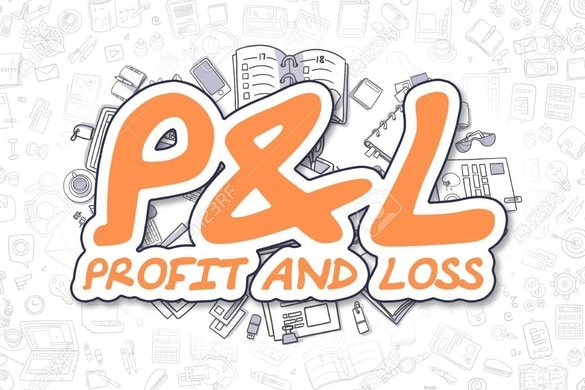# Household Arithmetic Relating to Profit, Interest, Discount and Commission

Welcome to Class !!

In today’s Mathematics class, We will be discussing “Simple Interest. We will so be looking at Profit and Loss. We will ten round up with Discount and Commision. We hope you enjoy the class!### SIMPLE INTEREST

Interest is the money paid for saving a particular amount of money.

Simple interest can be calculated using the formula

Where P= principal, R=rate & T= time

Also total amount =principal +interestExample 1

Find the simple interest on N60, 000 for 5 years at 9% per annum

Solution

Applying

where P= N 60,000 R=9% T=5 years

Example 2

A man borrows N 1,600,000 to buy a house. He is charged interest at the rate of 11% per annum. In the first year, he paid the interest on the loan. He also paid back N 100,000 of the money borrowed. How much did he pay back altogether? If he paid this money by monthly instalment, how much did he pay per month?

Solution

P= N 1,600,000   R=11% T=1 year

Interest on N 1,600,000

The total amount paid in the first year= N 100,000 + N 176,000 = N 276,000

Monthly payment =

$\frac{276,000}{12}$

= N 23,000

Classwork

1. Find the simple interest on the following:
• N 10,000 for 31/2 years at 4% per annum
• N 20,000 for 4 years at 4% per annum
2. A man got N 1,800,000 loan to buy a house. He paid interest at a rate of 9% per annum. In the first year, he paid the interest on the loan. He also paid back N 140,000 of the money he borrowed. (a) How much did he pay in the first year altogether?#### Profit and loss percent

Profit means to gain, while loss is the inverse of profit.

% Profit is the percentage of the gain made from a particular product or item

To find the selling price of an article

At loss, Selling price = cost price – loss

At gain, Selling = cost price + profit

Example

1. A trader buys a kettle for N 800 and sells at a profit of 15%. Find the actual profit and the selling price

Solution

Profit =15 of N 800

=15 x800

= N 120

Selling price = C. + profit

= 800+120

= N 920

1. A hat is bought for N 250 and sold for N 220, what is the loss percentage

Solution

Cost price C.P = N 250

Selling price = N 220

Lost= N 250- N 220 = N 30

% Loss =

Classwork

1. Find the actual profit and the selling price of a material which cost #1000 sold at a profit of 15%
2. A farmer buys a piece of land for #40,000 and sells it for N 33,000 what is the percentage loss?
3. A car that cost N 336,000 was sold at a loss of 17½%. What is the selling price

#### Discount and Commission

A discount is a reduction in the price of goods or items. Discounts are often given for paying in cash.

Commission: this is a payment or reward for selling an item.

Examples

• Find the discount price, if a discount of 25% is given on a market price of #9,200

Solution

Discount =25% of N 9,200

• A radio cost N 5,400. A 12½% discount is given for cash. What is the cash price

Solution

Discount =12 ½% of N 5,400

• A bank charges 2½   % commission for issuing a bank draft to its customers, if a customer obtained a bank draft for N 84,000 from the bank, calculates the total cost of the bank draft.

Solution

Commission =22% of N 84,000

5/2/100 x 84,000

=5/2×100 x84, 000

= N 2,100

Total cost of bank draft = 84,000+ N 2,000 = N 86,100

Classwork

1. Find the discount price if a discount of 20% is given on a market of N 2,915
2. The selling price of a table is N 14,000, the trader gives a 25% discount for cash, what is the cash price
3. An insurance agent sells N 284,000 worth of insurance, his commission is 20%. How much money does he get?

New General Mathematics, UBE Edition, Chapter 1, pages 78-79

Essential Mathematics by A J S Oluwasanmi, Chapter 1, pages 61-64

WEEKEND ASSIGNMENT

1. Calculate the simple interest on N 200 in 2 years at 4% per annum (a) N 160n (b) N 240 (c) N 16 (d) N 260
2. Calculate the simple interest on N 20,000 for 21/2 years at 2% per annum (a) N 10 (b) N10, 000  (c) N 1000 (d) N 2000
3. Find the simple interest on N 40,000 for 1 year at 5% per annum (a) N 100 (b) N 250 (c) N 2,590 (d) N 50
4. What is the simple interest on #70,000 for 1 year at 4% per annum (a) N 2,800 (b) N 2,000 (c) N 2,400  (d) N 2,300
5. Find the simple interest on N 10,000 for 3 years at 6% per annum (a) N 1,800 (b) N 1000 (c) N1,850 (d) N 1,200

THEORY

1. Find the simple interest on the following
• N 55,000 for 4 years at 60% per annum
• N 25,000 for 3 years at 5% per annum

We have come to the end of this class. We do hope you enjoyed the class?

Should you have any further question, feel free to ask in the comment section below and trust us to respond as soon as possible.

In our next class, we will be talking about Approximation of Numbers. We are very much eager to meet you there.